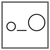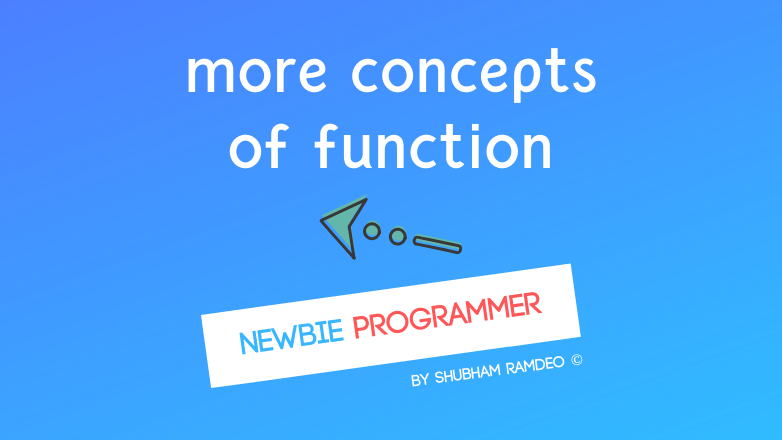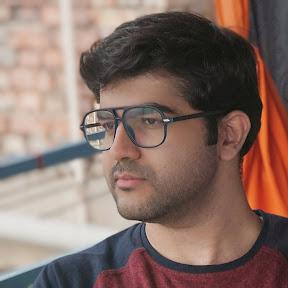Fuddlepixel coz tech is fun();

# More Concepts of Functions

Let us discuss some more advanced stuffs of using functions and some tricks and concepts to make programming style better in C language.

By Shubham Ramdeo
09 October 2015 · 6 mins read
# `c`  `newbie`That’s are the important programming concepts involving functions !

Welcome to the Newbie Programmer Series ! In the last post, we had a basic discussion about functions. Now we know how to declare a function and how to use them. You may revise the previous one (click here). In this post we will discuss some advanced things regarding functions and their usage. So if you are new to this series, please go to index (click here) and read out all the previous parts so that you can easily understand what we are doing down below.

## Non Returning or Void Functions

Heading looks awkward ? Its easy. I have not discussed the void functions in the previous post so I am doing it here. Void or Non Returning Functions are the functions which are not involving and returning any special type of variable.

When we made the add function in the previous post, it was returning the sum of two variables. This sum is an integer so the function become an integer function.

But what about a function like this :

``````say_hello()
{
printf("Hello Everyone ! \n");
}
``````

This function when used will simply print the message “Hello Everyone !” on the screen. It is involving no variables. And it’s returning no variables. The role of the function completes when the message is printed. Such a function is a void function. void means no property. So as the function is having no variable type, it is a void function.

To declare a void function, just write void. Usage is similar to int, float, char etc.

So the above function must be declare as :

``````void say_hello()
{
printf("Hello Everyone ! \n");
}
``````

## main Function

A program may have too many functions inside it. But which function will start first ? Its simple, the main() function starts and follows it and all the other functions are skipped if they are not used.

So,

``````#include <stdio.h>
main()
{
}

void say_hello()
{
printf("Hello Everyone ! \n");
}
``````

Will do nothing !

But this one will do:

``````#include <stdio.h>
main()
{
say_hello();
}

void say_hello()
{
printf("Hello Everyone ! \n");
}
``````

You may have a doubt that Can the main() function have data types as we are using void, int etc for other functions, is it true for main() true ? And the answer is YES. It does have. I have just ignored it as we are not using command line arguments (learn later).

So in the above function, It is better to replace :

main() by void main()

In case it involves numbers, you can use int main() as well.

## Using Functions

I have already discussed it in the previous post but let’s go deeper. Functions can be used anywhere. A function inside a function. Like we did with nested if else (click here) Or alone in main function, whatever you want but lets discuss some basic ways :

#### Type A

The simple void functions like :

``````void say_hello()
{
printf("Hello Everyone ! \n");
}
``````

Can be used anywhere simply as :

``````say_hello();
``````

with semicolon.

#### Type B

If a function is involving some parameters but returning nothing like :

``````void fav_num( int num )
{
printf("Do you know %d is my favorite number ! \n", num);
}
``````

Again it can be used simply like as fav_num(4);

#### Type C

But if the function is returning some value like :

The following function calculates the square for the given number

``````int square( int num )
{
return ( num * num )
}
``````

Then we can use this in two ways. Suppose we want to print square of 4 then inside the main() function :

#### First Way :

``````sqr = square(4);
printf("%d", sqr);
``````

#### Second Way :

``````printf("%d", square(4) );
``````

If that’s only what you want you are to do, Second way is better as we have skipped making an another variable sqr.

## Recursion

Recursion is like using the same function within itself. Well the definition is hard, so its easy to learn it practically.

Lets make a program to calculate factorial. Factorial means product of all numbers until 1. Suppose if I enter 5, It must give me the product 54321. If I entered 11, It must give the product 1110987654321.

Now first see the code. Then we will discuss how it works.

``````#include<stdio.h>
int main()
{
int n;
printf("Enter a number  \n");
scanf("%d", &n);
printf("The Answer is : %d \n", fact(n));
return 0;
}

int fact(int n)
{
if( n != 1 )
{
return ( n * fact(n-1) );
}
}
``````

OUTPUT :

``````Enter a number
6
``````

WORKING :The main function is simple, it asks a number then print the message along with the factorial which will be using the fact function.

Now the fact function, our main focus. What we need to calculate on giving 6 is 654321. We do not want 654321* -1* -2… We have to end it at ‘1’. Even the 1 is useless as any number multiplied by 1 is itself so we can ignore multiplying by 1. So we have used the if which is meaning that “calculate factorials if n is not 1” As soon as the n go 1, the function stops.

Now in the fact function, It is returning the number n and factorial of n-1. The computer do the following steps in the fact function :

``````fact(6)
6 * fact(5) //note n * fact(n-1)
6 * 5 * fact (4)
6 * 5 * 4 * fact (3)
6 * 5 * 4 * 3 * fact (2)
6 * 5 * 4 * 3 * 2 * fact (1)
Oh now n = 1 The function stops.
``````

Now the function stops and is having the final value : 6 * 5 * 4 * 3 * 2

Which is 720. And this value is used by the main function to print the answer.

That’s called recursion but we will do many better examples like Fibonacci Numbers, Summations and even some practical ones as we did here : Some Practical Applications of Control Flow (click here)

I think we have done enough for today, you may play some more. While we will do more interesting stuffs in the next part. So stay connected.

##### Update me weekly ✉

Subscribe below and we’ll send you a weekly email summary of all new Code tutorials. Never miss out on learning about the next big thing.

#### Shubham RamdeoShubham Ramdeo is the founder of Fuddlepixel. He is a geek, a writer, involved with many technical and humanity projects.

Meet me!

#### Recent#### Related

`c`

`newbie`

Top